编译原理实验_编译原理实验一 - CSDN

• ## 编译原理实验：词法分析

万次阅读 多人点赞 2020-07-06 09:44:07
编译原理实验：词法分析1. 实验题目：词法分析实验目的实验内容实验要求输入输出2. 设计思想3.算法流程4. 源程序5. 调试数据 1. 实验题目：词法分析 实验目的 根据PL/0语言的文法规范，编写PL/0语言的词法分析...
编译原理实验：词法分析1. 实验题目：词法分析实验目的实验内容实验要求输入输出2. 设计思想3.算法流程4. 源程序5. 调试数据
1. 实验题目：词法分析
实验目的

根据PL/0语言的文法规范，编写PL/0语言的词法分析程序；或者调研词法分析程序的自动生成工具LEX或FLEX，设计并实现一个能够输出单词序列的词法分析器。
通过设计调试词法分析程序，实现从源程序中分出各种单词的方法；加深对课堂教学的理解；提高词法分析方法的实践能力。
掌握从源程序文件中读取有效字符的方法和产生源程序的内部表示文件的法。
掌握词法分析的实现方法。上机调试编出的词法分析程序。

实验内容
已给PL/0语言文法，输出单词符号（关键字、专用符号以及其它标记）。
实验要求

把词法分析器设计成一个独立一遍的过程。
词法分析器的输出形式采用二元式序列，即：(单词种类,单词的值)

输入输出
输入：
const a=10;
var b,c;
begin
c:=a+b;
write( c);
end.
输出：
(constsym, const)
(ident , a)
(eql, =)
(number, 10)
(semicolon, ; )
(varsym, var)
(ident,b)
(comma, ,)
(ident, c)
(semicolon, ; )
(beginsym, begin)
(lparen,( )
(ident, b)
(rparen, ))
(semicolon, ; )
(ident, c)
(becomes, := )
(ident,  a)
(plus,  +)
(ident,b )
(semicolon, ; )
(writesym,write)
(lparen, ( )
(ident, c)
(rparen,) )
(endsym, end )
(period, .)
2. 设计思想
基本字：

单词（编码）
正规式r

begin(beginsym)
begin

call(callsym)
call

const(constsym)
const

do(dosys)
do

end(endsym)
end

if(ifsym)
if

odd(oddsym)
odd

procedure(proceduresym)
procedure

var(varsym)
var

while(whilesym)
while

write(writesym)
write

then(thensym)
then

标识符：

单词（编码）
正规式r

<标识符>(ident)
（字母)(字母 |数字)*

常数：

单词（编码）
正规式r

<常数>(ident)
（数字)(数字)*

运算符：

单词（编码）
正规式r

+(plus)
+

-(minus)
-

*(times)
*

/(slash)
/

=(eql)
=

<>(neq)
<>

<(lss)
<

<=(leq)
<=

>(gtr)
>

>=(geq)
>=

:=(becomes)
:=

界符：

单词（编码）
正规式r

(  (lparen)
(

)  (rparen)
)

,  (comma)
,

;  (semicolon)
;

.  (period)
.

3.算法流程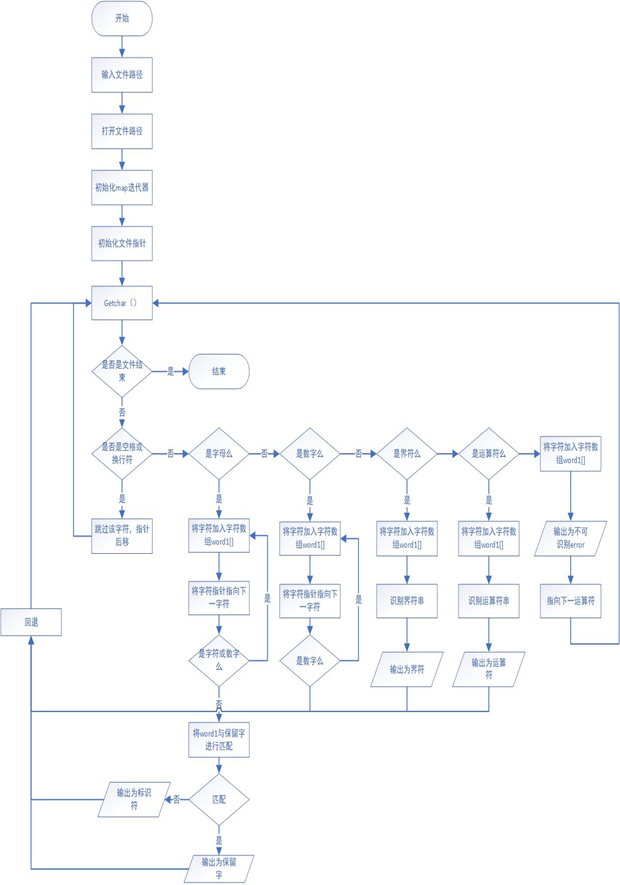词法分析程序打开源文件，读取文件内容，直至遇上文件结束符，然后读取结束。
接下来就要对源文件从头到尾进行扫描了，从头开始扫描，这个时候扫描程序首先要询问当前的字符是不是空格，若是空格，则继续扫描下一个字符，直至不是空格。然后询问这个字符是不是字母，若是则进行标识符和保留字的识别；若这个字符为数字，则进行数字的判断。否则，依次对这个字符可能的情况进行判断（界符和运算符），若将所有可能都走了一遍还是没有知道它是谁，则认定为错误符号，输出该无法识别error，程序结束。每次成功识别了一个单词后，单词都会存在word1[]数组中，然后字符指针往后移，进行下一个单词的识别。
主控程序需要负责对每次识别的种别码进行判断，对于不同的单词种别做出不同的反应，直至文件结束。
本次实验我采用了map这个STL关联容器，主要是考虑到词法分析中的数据映射的关系，因此采用这种结构。map提供一对一的数据处理能力，其中第一个可以称为关键字，每个关键字只能在map中出现一次，第二个可能称为该关键字的值。这个容器是非常方便使用的，对于查找可以直接使用迭代器进行，利用find（）函数，若一直到末尾都未找到，则是不能识别或为标识符。

4. 源程序
#include<bits/stdc++.h>
using namespace std;
map<string,string> word;//应用map数据结构形成一个string->string的对应
std::map<string,string>::iterator it;//用来遍历整个对应关系的迭代器
void map_init(){//对应关系进行初始化
word["begin"]="beginsym";
word["call"]="callsym";
word["const"]="constsym";
word["do"]="dosym";
word["end"]="endsym";
word["if"]="ifsym";
word["odd"]="oddsym";
word["procedure"]="proceduresym";
word["then"]="thensym";
word["var"]="varsym";
word["while"]="whilesym";
word["write"]="writesym";
word["+"]="plus";
word["-"]="minus";
word["*"]="times";
word["/"]="slash";
word["="]="eql";
word["<>"]="neq";
word["<"]="lss";
word["<="]="leq";
word[">"]="gtr";
word[">="]="geq";
word[":="]="becomes";
word["("]="lparen";
word[")"]="rparen";
word[","]="comma";
word[";"]="semicolon";
word["."]="period";
}
int main(){
map_init();//初始化
char ch;
char a;
string word1;//string变量识别单词
string str;//string变量进行字符识别
ifstream infile("F:\\编译原理\\第一次实验\\analysis.txt");//文件输入流
ofstream outfile("F:\\编译原理\\第一次实验\\result.txt");//文件输出流
ostringstream buf;
while(buf&&infile.get(ch)) buf.put(ch);//将文件中的字符读出来
str= buf.str();//将得到的字符储存到string类型变量中
int csize=str.length();
for(int i=0;i<csize;i++){//对整个字符串进行遍历
while(str[i]==' '||str[i]=='\n') i++;//若最开始为空格或换行符，则将指针的位置往后移
if(isalpha(str[i])){//对标识符和基本字进行识别,调用库函数isalpha()
word1=str[i++];
while(isalpha(str[i])||isdigit(str[i])){
word1+=str[i++];
}
it=word.find(word1);
if(it!=word.end()){//判断是不是基本字，若为基本字则进行输出
cout<<"("<<word[word1]<<","<<word1<<")"<<endl;
}
else{//否则直接输出
cout<<"(ident"<<","<<word1<<")"<<endl;
}
i--;
}
else if(isdigit(str[i])){//判断是不是常数，调用库函数isdigit()
word1=str[i++];
while(isdigit(str[i])){
word1+=str[i++];
}
if(isalpha(str[i])){
cout<<"error!"<<endl;
break;
}
else{
cout<<"(number"<<","<<word1<<")"<<endl;
}
i--;
}else if(str[i]=='<'){//对<,<=分别进行判断
word1=str[i++];
if(str[i]=='>'){
word1+=str[i];
cout<<"("<<word[word1]<<","<<word1<<")"<<endl;
}else if(str[i]=='='){
word1+=str[i];
cout<<"("<<word[word1]<<","<<word1<<")"<<endl;
}else if(str[i]!=' '||!isdigit(str[i])||!isalpha(str[i])){
cout<<"("<<word[word1]<<","<<word1<<")"<<endl;
}else{
cout<<"error!"<<endl;
break;
}
i--;
}else if(str[i]=='>'){//对>,>=分别进行判断
word1=str[i++];
if(str[i]=='='){
word1+=str[i];
cout<<"("<<word[word1]<<","<<word1<<")"<<endl;
}else if(str[i]!=' '||!isdigit(str[i])||!isalpha(str[i])){
cout<<"("<<word[word1]<<","<<word1<<")"<<endl;
}else{
cout<<"error!"<<endl;
break;
}
i--;
}else if(str[i]==':'){//对:=进行判断
word1=str[i++];
if(str[i]=='='){
word1+=str[i];
cout<<"("<<word[word1]<<","<<word1<<")"<<endl;
}else{
cout<<"error!"<<endl;
break;
}
i--;
}else{//对其他的基本字依次进行判断
word1=str[i];
it=word.find(word1);
if(it!=word.end()){
cout<<"("<<word[word1]<<","<<word1<<")"<<endl;
}else{
cout<<"error!"<<endl;
break;
}
}
}
infile.close();
return 0;
}

5. 调试数据
待输入的文件流：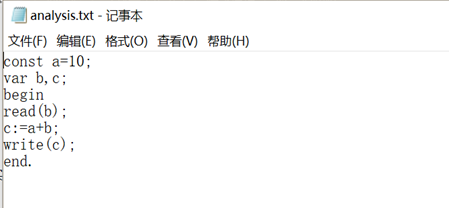输出数据：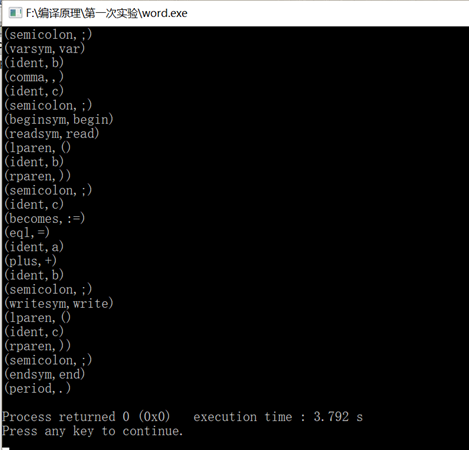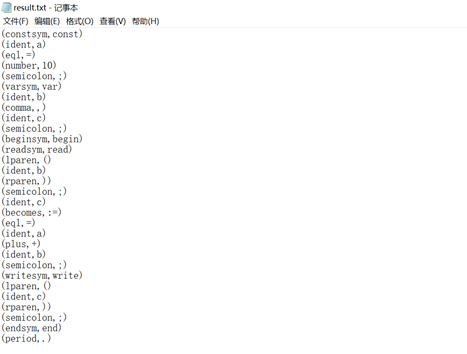说明：如上实验仅符合当时实验要求的相关条件，其他的需求略微进行更改就行，思想是一样的，还是很简单的。输入输出自己按自己需求更改即可。


展开全文编译原理实验
• ## 编译原理实验一 词法分析器

万次阅读 多人点赞 2018-07-03 15:51:15
编写一个词法分析程序
编写一个词法分析程序实验目的：理解词法分析在编译程序中的作用；                 加深对有穷自动机模型的理解；                 掌握词法分析程序的实现方法和技术。实验内容：选择部分C语言的语法成分，设计其词法分析程序，要求能够识别关键字、运算符、分界符、标识符、常量（至少是整型常量，可以自己扩充识别其他常量）等，并能处理注释、部分复合运算符（如>=等）。实验要求：（1）待分析的简单的语法     关键字：begin   if   then   while   do    end     运算符和界符：:=   +   -   *   /   <   <=   >   >=   <>   =   ;   (   )   #     其他单词是标识符id和整型常数num，通过以下正规式定义：     id=l(l|d)*     num=dd*     空格、注释：在词法分析中要去掉。（2）各种单词符号对应的种别编码        单词符号  种别码  单词符号  种别码 begin  1  \0  1000 if  2  (  26 then  3  )  27 while  4  [  28 do  5  ]  29 end  6  {  30 int  7  }  31 main  8  ,           32 return  12  :  33 cout  13  ;  34 l（l|d）*  10  >   35 :=  18  <   36 dd*  20  >=  37 ==  21  <=  38 +  22  !=  40 -  23  “  41 *  24  #  0 /  25  !  -1该程序实现词法分析，从文件data.txt中读取一段小程序，分解出一个个的单词，其中有关键词，有界符、运算符等等，代码还需实现去掉空格、回车、注释等等情况，最后的输出结果是以单词二元组（单词种别码，单词自身的值）的形式输出。主要的函数有：char m_getch()   从输入缓冲区读取一个字符到ch中void getbc( )     去掉空白字符void concat( )     拼接单词int letter( )       判断输入字符是否是字母int digit( )        判断输入字符是否是数字int reserve( )     检索关键字表格，判断单词是否为关键字void retract( )     回退一个字符WORD * scanner( )  词法扫描程序，返回值是二元组在词法扫描程序中，扫描一个个字符，去掉空白，判断是否为注释等等。程序代码如下：#include <iostream>
#include <cstdio>
#include <cstring>
#include <cstdlib>
#define _KEY_WORDEND "waiting for your expanding"
using namespace std;
typedef struct //词的结构，二元组形式（单词种别，单词自身的值）
{
int typenum; //单词种别
char * word;
}WORD;
char input;
char token = "";
int p_input; //指针
int p_token;
char ch;
char * rwtab[] = { "begin","if","then","while","do","end","int","main",
"else","float","double","return","cout",_KEY_WORDEND };

WORD * scanner();//扫描

int main()
{
int over = 1;
WORD* oneword = new WORD;

//实现从文件读取代码段
cout << "read something from data.txt" << endl;
FILE *fp;
if((fp=freopen("data.txt","r",stdin))==NULL)
{
return 0;
}
else
{
while ((scanf("%[^#]s", &input)) != EOF)
{
p_input = 0;
while (over < 1000 && over != -1)
{
oneword = scanner();
if (oneword->typenum < 1000)
{
if(oneword->typenum != 999)
cout << "[  "<< oneword->typenum <<"\t"<< oneword->word <<"  ]"<< endl;
}
over = oneword->typenum;
}
scanf("%[^#]s", input);
}
}
return 0;
}

//从输入缓冲区读取一个字符到ch中
char m_getch()
{
ch = input[p_input];
p_input++;
return ch;
}

//去掉空白符号
void getbc()
{
while (ch == ' ' || ch == 10)
{
ch = input[p_input];
p_input++;
}
}

//拼接单词
void concat()
{
token[p_token] = ch;
p_token++;
token[p_token] = '\0';
}

//判断是否字母
int letter()
{
if (ch >= 'a'&&ch <= 'z' || ch >= 'A'&&ch <= 'Z')
return 1;
else
return 0;
}

//判断是否数字
int digit()
{
if (ch >= '0'&&ch <= '9')
return 1;
else
return 0;
}

//检索关键字表格
int reserve()
{
int i = 0;
while(strcmp(rwtab[i], _KEY_WORDEND))
{
if (!strcmp(rwtab[i], token))
return i + 1;
i++;
}
return 10;//如果不是关键字，则返回种别码10
}

//回退一个字符
void retract()
{
p_input--;
}

//词法扫描程序
WORD * scanner()
{
WORD * myword = new WORD;
myword->typenum = 10;  //初始值
myword->word = "";
p_token = 0;   //单词缓冲区指针
m_getch();
getbc();//去掉空白

if (letter())//判断读取到的首字母是字母
{
//如int
while (letter() || digit())
{
concat(); //连接
m_getch();
}
retract(); //回退一个字符
myword->typenum = reserve();//判断是否为关键字，返回种别码
myword->word = token;
return myword;
}
else if (digit())  //判断读取到的单词首字符是数字
{
while (digit()) //所有数字连接起来
{
concat();
m_getch();
}
retract();
//数字单词种别码统一为20，单词自身的值为数字本身
myword->typenum = 20;
myword->word = token;
return(myword);
}
else switch (ch)
{
case '=':
m_getch();//首字符为=,再读取下一个字符判断
if (ch == '=')
{
myword->typenum = 39;
myword->word = "==";
return(myword);
}
retract();//读取到的下个字符不是=，则要回退，直接输出=
myword->typenum = 21;
myword->word = "=";
return(myword);
break;
case '+':
myword->typenum = 22;
myword->word = "+";
return(myword);
break;
case '-':
myword->typenum = 23;
myword->word = "-";
return(myword);
break;
case '/'://读取到该符号之后，要判断下一个字符是什么符号，判断是否为注释
m_getch();//首字符为/,再读取下一个字符判断
if (ch == '*') // 说明读取到的是注释
{
m_getch();

while(ch != '*')
{
m_getch();//注释没结束之前一直读取注释，但不输出
if(ch == '*')
{
m_getch();
if(ch == '/')//注释结束
{
myword->typenum = 999;
myword->word = "注释";
return (myword);
break;
}
}

}

}
else
{
retract();//读取到的下个字符不是*，即不是注释，则要回退，直接输出/

myword->typenum = 25;
myword->word = "/";
return (myword);
break;
}
case '*':
myword->typenum = 24;
myword->word = "*";
return(myword);
break;
case '(':
myword->typenum = 26;
myword->word = "(";
return(myword);
break;
case ')':
myword->typenum = 27;
myword->word = ")";
return(myword);
break;
case '[':
myword->typenum = 28;
myword->word = "[";
return(myword);
break;
case ']':
myword->typenum = 29;
myword->word = "]";
return(myword);
break;
case '{':
myword->typenum = 30;
myword->word = "{";
return(myword);
break;
case '}':
myword->typenum = 31;
myword->word = "}";
return(myword);
break;
case ',':
myword->typenum = 32;
myword->word = ",";
return(myword);
break;
case ':':
m_getch();
if (ch == '=')
{
myword->typenum = 18;
myword->word = ":=";
return(myword);
break;
}
else
{
retract();
myword->typenum = 33;
myword->word = ":";
return(myword);
break;
}
case ';':
myword->typenum = 34;
myword->word = ";";
return(myword);
break;
case '>':
m_getch();
if (ch == '=')
{
myword->typenum = 37;
myword->word = ">=";
return(myword);
break;
}
retract();
myword->typenum = 35;
myword->word = ">";
return(myword);
break;
case '<':
m_getch();
if (ch == '=')
{
myword->typenum = 38;
myword->word = "<=";
return(myword);
break;
}
else if(ch == '<')
{
myword->typenum = 42;
myword->word = "<<";
return(myword);
break;
}
else
{
retract();
myword->typenum = 36;
myword->word = "<";
return (myword);
}
case '!':
m_getch();
if (ch == '=')
{
myword->typenum = 40;
myword->word = "!=";
return(myword);
break;
}
retract();
myword->typenum = -1;
myword->word = "ERROR";
return(myword);
break;
case ' " ':
myword->typenum = 41;
myword->word = " \" ";
return(myword);
break;
case '\0':
myword->typenum = 1000;
myword->word = "OVER";
return(myword);
break;
case '#':
myword->typenum = 0;
myword->word = "#";
return (myword);
break;
default:
myword->typenum = -1;
myword->word = "ERROR";
return(myword);
break;
}
}

代码运行结果如下：其中标注为1的部分是从data.txt中读取到的小程序，程序输出在小程序下方：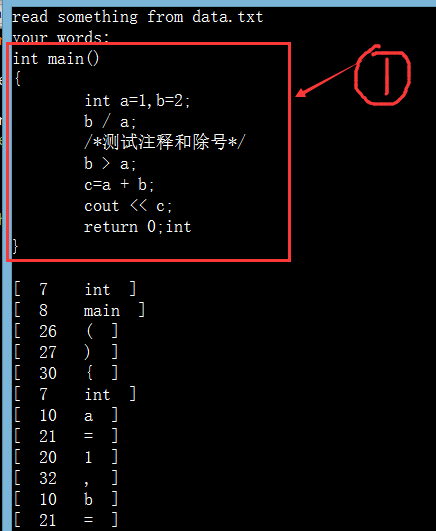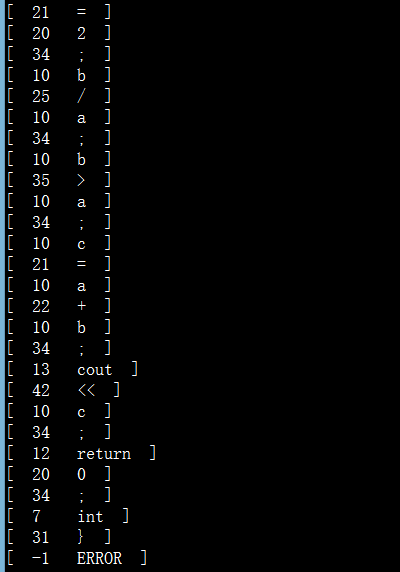展开全文编译原理 实验 词法分析程序
• ## 编译原理实验

千次阅读 2018-10-13 17:10:04
一、实验目的 学习使用词法分析程序自动构造工具 Flex 熟悉LEX源程序语法 掌握词法分析程序的自动构造方法 二、实验平台 Windows + Flex 三、基础内容 实现以下步骤, 掌握Flex的工作过程 i. 构造 LEX 源程序...
一、实验目的

学习使用词法分析程序自动构造工具 Flex

熟悉LEX源程序语法

掌握词法分析程序的自动构造方法
二、实验平台
Windows + Flex
三、基础内容

实现以下步骤, 掌握Flex的工作过程
i.  构造 LEX 源程序, 例如命名为 Test.Lex
ii.  编译 LEX 源程序, 生成C语言词法分析程序 lex.yy.c, 步骤如下:
在DOS命令提示符下执行编译   flex  Test.Lex 得到目标文件   lex.yy.c
iii.  在VC中编译 lex.yy.c，产生可执行程序 lex.yy.exe
iv.  运行生成的可执行文件 lex.yy 或  lex.yy  < InputFile

测试目录 SRC_FLEX中的范例程序,了解其功能及实现。

四、附加内容
★★ 输入一个 C源程序文件, 用FLex 实现以下任务
a)  添加行号。
b)  将文件中每个非空的空白符号序列替换为单个空格。
c)  将文件中所有关键字转换为大写字母。
d)  将文件中所有标识符转换为小写字母，且以下划线开头。
e)  将文件中所有指数形式的常量转换为小数形式。
例如：  2.5e3  被转换为  2500
3.7e-2    被转换为    0.037
f)  将转换后的文件存入另一个文件。
参考资料

LEX范例程序：SRC_FLEX

b.lex文件如下：

%{
#include <stdio.h>
FILE *f1;
FILE *f2;
int hanghao=2;
%}

keyword  auto|break|case|char|const|continue|default|do|double|else|enum|extern|float|for|goto|if|int|long|register|return|short|signed|sizeof|static|struct|switch|typedef|union|unsigned|void|volatile|while|AUTO|BREAK|CASE|CHAR|CONST|CONTINUE|DEFAULT|DO|DOUBLE|ELSE|ENUM|EXTERN|FLOAT|FOR|GOTO|IF|INT|LONG|REGISTER|RETURN|SHORT|SIGNED|SIZEOF|STATIC|STRUCT|SWITCH|TYPEDEF|UNION|UNSIGNED|VOID|VOLATILE|WHILE
id       [A-Za-z]+
digit    [0-9]
huan     {digit}+(\.{digit}+)?(E[+-]?{digit}+)?

%%

[ ]+     {fprintf(f1," ");}

\n 	{fprintf(f1,"%s%u",yytext,hanghao++);}

{keyword} {
int i;
for(i=0;i<yyleng;i++)
{
if(yytext[i]>='a'&&yytext[i]<='z')
yytext[i]-=32;
}
fprintf(f1,"%s",yytext);
}

{id}      {
int i;
for(i=0;i<yyleng;i++)
{
if(yytext[i]>='A'&&yytext[i]<='Z')
yytext[i]+=32;
}
fprintf(f1,"_%s",yytext);
}

{huan}     {   float  t=0;
t=atof(yytext);
fprintf(f1,"%f",t);
}
.        fprintf(f1,"%s",yytext);
%%

int yywrap(void)
{
return 1;
}

main()
{
f1=fopen("f1.txt","w+");
fprintf(f1,"1");
yylex();
fclose(f1);
f1=fopen("f1.txt","r");
f2=fopen("f2.txt","w");
char e;
while((e=fgetc(f1) )!= EOF )
{fputc(e,f2);}
fclose(f1);
fclose(f2);
}



一、实验目的

学习使用词法分析程序自动构造工具Flex

熟悉LEX源程序语法

掌握词法分析程序的自动构造方法

二、实验平台
Windows+ Flex
三、基础内容

实现以下步骤, 掌握Flex的工作过程

i.         构造 LEX 源程序, 例如命名为 Test.Lex
ii.        编译 LEX 源程序, 生成 C 语言词法分析程序 lex.yy.c, 步骤如下:
在 DOS 命令提示符下执行编译  flex  Test.Lex 得到目标文件 lex.yy.c   iii. 在 VC 中编译lex.yy.c，产生可执行程序lex.yy.exe
iv. 运行生成的可执行文件 lex.yy 或 lex.yy  < InputFile

测试目录SRC_FLEX中的范例程序,了解其功能及实现。

四、附加内容
输入一个 C 源程序文件, 用 FLex 实现以下任务
a)        添加行号。
b)        将文件中每个非空的空白符号序列替换为单个空格。
c)        将文件中所有关键字转换为大写字母。
d)        将文件中所有标识符转换为小写字母，且以下划线开头。
e)        将文件中所有指数形式的常量转换为小数形式。
例如：        2.5e3被转换为   2500                 3.7e-2  被转换为        0.037 f) 将转换后的文件存入另一个文件。
声明部分
%%
转换规则
%%
辅助函数
Lex程序的每个转换规则的形式如下：
模式{动作}
%{%} 括号中间的所有内容将直接复制到lex.yy.c中。
一个lex程序的结构如下：
yytext 是一个指向词素开头的指针
yyleng存放刚找到的词素的长度
源代码：
Demo.lex
%{
#include<stdio.h>
FILE* f1;
int n =1;
%}

keywords auto|break|case|char|const|continue|defaultdo|double|else|enum|extern|float|for|goto|if|int|long|register|return|short|signed|sizeof|static|struct|switch|typedef|union|unsigned|void|volatile|while
id [a-zA-Z][a-zA-Z0-9]*
number [0-9]
enumber {number}+(\.{number}+)?e([+-])?{number}+
space [\ \t]+

%%

{space} {fprintf(f1," ");}

{keywords} {
int i = 0;
while(i<yyleng && yytext[i]>='a' && yytext[i]<='z')
{
yytext[i]-=32;
i++;
}
fprintf(f1,"%s",yytext);
}

{id} {
int i =0 ;
while(i<yyleng && yytext[i]>='A' && yytext[i]<='Z')
{
yytext[i]+=32;
i++;
}
fprintf(f1,"_%s",yytext);
}

{enumber} {
float f = atof(yytext);
fprintf(f1,"%f",f);
}

[^\n] {fprintf(f1,"%s",yytext);}
\n {
fprintf(f1,"%s%5d  ",yytext,n++);
}

%%

int yywrap(void)
{
return 1;
}

main()
{
f1 = fopen("demo.txt","a+");
fprintf(f1,"%5d  ",n++);
yylex();
fprintf(f1,"\n");
fclose(f1);
}


运行：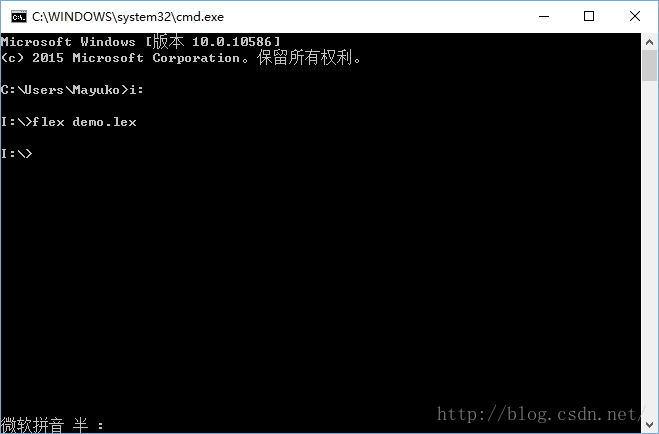将生成的lex.yy.c编译
用lex.yy.exe 打开一个文件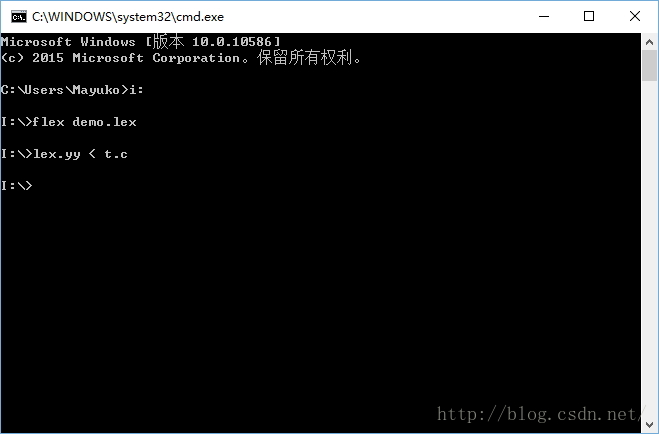规则化的文件：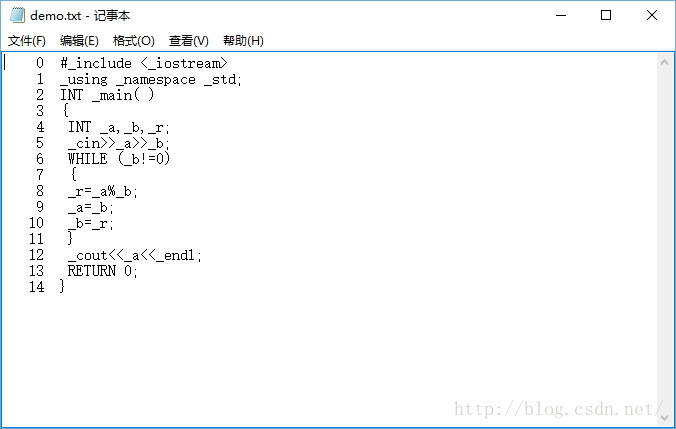展开全文实验
• 大三上学期的编译原理实验，自己用C#写的代码。有词法分析、LL1分析、LR1分析这三次实验。
• 版权声明：本文为原创文章，版权归 Geekerstar 所有。 ... 除了有特殊标注文章外欢迎转载，但请务必标明出处，格式如上，谢谢合作。 1 概述 ...通过某种高级语言（如C/...2 实验目标 理解并掌握词法，语法分析的...

版权声明：本文为原创文章，版权归 Geekerstar 所有。

本文链接：http://www.geekerstar.com/technology/105.html

除了有特殊标注文章外欢迎转载，但请务必标明出处，格式如上，谢谢合作。

1 概述

通过某种高级语言（如C/C++，Java）实现词法，语法分析器的功能。

2 实验目标

理解并掌握词法，语法分析的原理与方法。
能够使用某种语言实现词法，语法分析程序。
对编译的基本概念，原理和方法有完整和清楚的理解，并能正确而熟练的运用。
3 实验描述

3.1 实验要求

采用词法分析程序作为语法分析程序子程序的方法，实现词法、语法分析。

具体要求如下：

词法分析程序作为语法分析程序的子程序。
输入数据：程序段。
输出结果：语法分析结果，包括错误列表。
3.2 本实验概述

本次实验采用C++编写词法语法分析器，在此基础上，采用递归下降
分析程序，实现对词法分析程序所提供的单词序列的语法检查和结构分
析。PL/0语言功能简单，结构清晰，可读性强，而又具备了一般的高级
程序设计语言的必须部分，所以采用PL/0语言的编译程序能充分体现一
个高级语言编译程序实现的基本方法和技术，故采用PL/0语言进行词法 语法分析。

4 技术分析

4.1 词法语法分析

本次实验主要用到的是基本的数据结构分析，C++编程技术，编译原理等，
其中对编译的基本概念抓住词法分析与语法分析两个部分来理解：

词法分析
词法分析器根据词法规则识别出源程序中的各个记号，每个记号代表一
类单词。源程序中常见的记号可以归为几大类：关键字、标识符、字面量和
特殊符号。词法分析器的输入是源程序，输出是识别的记号流。词法分析器
的任务是把源文件的字符流转换成记号流。它是编译过程的第一个阶段。其
主要任务是从左到右依次描描字符串形式的源程序的各个字符，逐个识别出
其中的单词，并将其转换成为内部编码形式的单词符号串输出，用于进行语 法分析。

概括的说，语法器在其工作过程中，一般应完成下列的任务：

（1）识别出源程序中的各个单词符号，并将其转换成内部编码形式；

（2）删除无用的空白字符、回车字符以及其他非实质性字符；

（3）删除注释；

（4）进行词法检查，报告所发现的错误。

此外，视编译工作流程的组织，一些编译程序在进行词法分析时，还要
完成将所识别出的标志符登录到符号表的工作。

语法分析：
语法分析是编译过程的核心，分析的任务是根据语法规则分析源程序的
语法结构，并在分析过程中，对源程序进行语法检查，如果语法没有错误，
则给出正确的语法结构，为语义分析和代码生成做准备。

目前语法分析方法有多种多样，大致分为自顶而下和自底而上两大类。
自顶而下又分为LL（1）分析方法和递归下降分析方法。自底而上又分为简
单优先文法、算符优先文法、LR（K）分析方法。下面主要介绍自底而上的
LR（K）分析方法。

自底向上分析法，也称移进-归约分析法。它的实现思想是对输入符号串
自左向右进行扫描，并将输入符逐个移入一个后进先出栈中，边移入边分析，
一旦栈顶符号串形成某个句型的句柄时，(该句柄对应某产生式的右部)，就
用该产生式的左部非终结符代替相应右部的文法符号串，这称为移步归约。
重复这一过程直到归约到栈中只剩文法的开始符号时则为分析成功，也就确
认输入串是文法的句子。否则，分析失败，表示输入符号串不是文法的一个
句子，其中必定存在语法错误。

根据以上编译的相关知识结合C++编程语言和部分数据结构的知识来写 词法语法分析器。

5 设计与实现

5.1 设计思路

编制一个递归下降分析程序，实现对词法分析程序所提供的单词序列的
语法检查和结构分析。利用C++编写递归下降分析程序，并对PL/0语言进行
语法分析。核心思想就是，从开始状态开始，按照文法展开式，逐级进行状 态分析，直
到分析完毕，如果在此期间出现状态不匹配，即语法错误，停止 分析。当然
在实际的语法分析器要有错误恢复机制，以发现其他的语法错 误。即一次报
告多个语法错误。此外要想实现语法分析，必须先有词法分 析，用词法分析
的结果进行语法分析。

用扩充的BNF表示如下：

编制一个递归下降分析程序，实现对词法分析程序所提供的单词序列的
语法检查和结构分析。

利用C++编写递归下降分析程序，并对PL/0语言进行语法分析。

\<程序>：：=begin\<语句串>end

\<语句串>：：=\<语句>{；\<语句>}

\<语句>：：=\<赋值语句>

\<赋值语句>：：=ID：=\<表达式>

\<表达式>：：=\<项>{+\<项> | -\<项>}

\<项>：：=\<因子>{*\<因子> | /\<因子>

\<因子>：：=ID | NUM | （\<表达式>）

输入单词串，以“#”结束，如果是文法正确的句子，则输出成功信息，
打印“语法分析成功！”，否则输出“语法分析错误（错误原因）”。

例如：

输入 begin a:=4; b:=2*3; c:=a+b end #

输出 语法分析成功！

输入 x:=a+b*c end #

输出 缺少begin！

单词符号
种别编码
Begin
1
If
2
Then
3
While
4
Do
5
End
6
标识符
10
数字
20
+
13
-
14
*
15
/
16
:
17
:=
18
\<
20
\<>
21
\<=
22
>
23
>=
24
=
25
;
26
(
27
)
28
#
0
5.2 实现方法

本实验采用C++编码，其中主要编写了以下几个函数及功能：

Void cifa() //词法分析

Void fun_yufa() //判断语法是否有错误

Void fun_op() //处理运算符(\*和/)

Void exp() //处理运算符（+和-）

Void fun_yuju() //判断是否有语句错误(:=)

Void fun_end() //判断程序是否结束

Void yufa() //采用递归下降的语法分析

其中cifa()进行词法分析，调用yufa()用词法分析的结果进行语法分 析。图1 词法分析部分代码图2 语法分析部分实现函数

5.3 测试用例

项目/软件
词法语法分析器
程序版本
V1.0

功能模块名
词法语法分析模块
编制人
XX

用例编号
T1.0
编制时间
207.11.20

功能特性
词法语法定义分析判断

测试目的
判断词法语法是否正确

测试数据
1：begin a:=3;b:=2*4;c:=a+b;end # 2：a:=3;b:=2*4;c:=a+b; end # 3：begin a:=3;b:=2*4;c:=a+b; #

测试用例
操作描述
代码
期望结果
实际结果
测试状态
1
输入第一段代码
begin a:=3;b:=2*4;c:=a+b;end #
语法分析正确
语法分析正确
良好
2
输入第二段代码
a:=3;b:=2*4;c:=a+b; end #
缺少Begin
缺少begin
良好
3
输入第三段代码
begin a:=3;b:=2*4;c:=a+b; #
缺少结束符
缺少结束符
良好
5.4 实验结果及分析

输入一段PL/0语言，比如输入：begin a:=3;b:=2*4;c:=a+b; end #

此段代码语法是正确的，所以经过词法语法分析输出的结果应该是：语
法分析正确！如下图所示：图3 实验结果1

当我们漏掉begin，语法分析器应该检测出并输出：缺少begin！如下图：图4 实验结果2

6 总结

经过这次实验，我对编译原理有了更近一步的理解，让我知道了词法分
析的功能是输出把它组织成单个程序，让我了解到如何设计、编写并调试词
法分析程序，对语法规则有明确的定义；编写的分析程序能够进行正确的语
法分析；对于遇到的语法错误，能够做出简单的错误处理，给出简单的错误
提示，保证顺利完成语法分析过程，并且通过实验的练习，加强了对基本概
念的理解和应用，对以后的学习也打下了基础。目前程序也存在着少量不足
之处，主要是语法分析部分还有不完善的地方，错误报告也有待改进，希望
在经过进一步的学习后，这些问题能逐步解决。

7 参考文献

1.互联网：百度，CSDN博客。

2.教材：《编译技术》张莉 高等教育出版社。

3.教材：C++ primer plus（第六版）

8 代码展示

#include "cstdio"
#include "string"
#include "iostream"
#include "algorithm"
#include "cstring"
using namespace std;

char str;            //从键盘输入
char bzf;      //判断是否是关键字
char ch;
char *keyword={"begin","if","then","while","do","end"};
int num,p,m,n,sum;
int x;

void cifa()   //词法分析
{
sum=0;
for(m=0;m<8;m++)
bzf[m++]=NULL;
m=0;
ch=str[p++];
while(ch==' ')       //去掉空格
ch=str[p++];
if(((ch<='z')&&(ch>='a'))||((ch<='Z')&&(ch>='A')))  //标识符
{
while(((ch<='z')&&(ch>='a'))||((ch<='Z')&&(ch>='A'))||((ch>='0')&&(ch<='9')))
{
bzf[m++]=ch;
ch=str[p++];
}
p--;
num=10;
bzf[m++]='\0';
for(n=0;n<6;n++)
if(strcmp(bzf,keyword[n])==0)
{
num=n+1;
break;
}
}
else if((ch>='0')&&(ch<='9'))  //数字
{
while((ch>='0')&&(ch<='9'))
{
sum=sum*10+ch-'0';
ch=str[p++];
}
p--;
num=11;
}
else
switch(ch)     //符号
{
case '<':
m=0;
ch=str[p++];
if(ch=='>')
{
num=21;
}
else if(ch=='=')
{
num=22;
}
else
{
num=20;
p--;
}
break;

case '>':
m=0;
ch=str[p++];
if(ch=='=')
{
num=24;
}
else
{
num=23;
p--;
}
break;

case ':':
m=0;
ch=str[p++];
if(ch=='=')
{
num=18;
}
else
{
num=17;
p--;
}
break;

case '+':
num=13;
break;

case '-':
num=14;
break;

case '*':
num=15;
break;

case '/':
num=16;
break;

case '(':
num=27;
break;

case ')':
num=28;
break;

case '=':
num=25;
break;

case ';':
num=26;
break;

case '#':
num=0;
break;

default:
num=-1;
break;
}
}
void term();
void exp();
void fun_yufa()   //判断语法是否错误
{
if((num==10)||(num==11))//关键字，数字
{
cifa();
}
else if(num==27)
{
cifa();
exp();

if(num==28)
{
cifa();          /*读下一个单词符号*/
}
else
{
printf("缺少‘(’\n");
x=1;
}
}
else
{
printf("语法错误\n");
x=1;
}
return;
}
void fun_op()  //处理运算符
{
fun_yufa();
while((num==15)||(num==16))//  '*'和'/'
{
cifa();             /*读下一个单词符号*/
fun_yufa();
}
return;
}

void exp()   //处理运算符
{
fun_op();
while((num==13)||(num==14))   //+和-
{
cifa();               /*读下一个单词符号*/
fun_op();
}

return;
}

void fun_yuju()  //判断是否有语句错误
{
if(num==10)
{
cifa();        /*读下一个单词符号*/
if(num==18)
{
cifa();      /*读下一个单词符号*/
exp();              }
else
{
printf("':='错误\n");
x=1;
}
}
else
{
printf("语法错误!\n");
x=1;
}

return;
}

void fun_end()  //判断程序结束的标志
{
fun_yuju();         /*调用函数statement();*/

while(num==26)
{
cifa();          /*读下一个单词符号*/
if(num!=6)
fun_yuju();          /*调用函数statement();*/
}

return;
}

void yufa()  //递归下降语法分析
{
if(num==1)
{
cifa();
fun_end();
if(num==6)
{
cifa();
if((num==0)&&(x==0))
printf("语法分析正确!\n");
}
else
{
if(x!=1) printf("缺少end!\n");
x=1;
}
}
else
{
printf("缺少begin!\n");
x=1;
}

return;
}

int main()
{
p=x=0;
printf("请输入一段语句以#结束: \n");
do
{
scanf("%c",&ch);
str[p++]=ch;
}while(ch!='#');
p=0;
cifa();
yufa();
return 0;
}


版权声明：本文为原创文章，版权归 Geekerstar 所有。

本文链接：http://www.geekerstar.com/technology/105.html

除了有特殊标注文章外欢迎转载，但请务必标明出处，格式如上，谢谢合作。

展开全文编译原理 词法分析器 语法分析
• ## 编译原理实验一:词法分析

万次阅读 多人点赞 2018-03-24 19:45:09
实验一：词法分析程序 一、实验目的 通过设计编制调试一个具体的词法分析程序，加深对词法分析原理的理解。并掌握在对程序设计语言源程序进行扫描过程中将其分解为各类单词的词法分析方法。 编制一个读单词过程，...编译原理
• 实验一（一） 基于C语言的TINY编译器与TM虚拟机（1学时） 实验目的 初步认识编译器系统和目标机，体会系统编程，了解条件编译和增量编程。 实验任务 获取TINY语言编译器相关源代码。 了解TINY语言定义，可...编译原理
• 词法、语法、语义分析器，最后有一个三合一版本。全部采用文件输入输出方式
• ## 编译原理实验报告

千次阅读 2018-06-27 15:29:51
1.2实验要求在掌握编译原理的基础上，对编译程序实例进行分析，通过编译程序的运行，检验编译程序输出结果的正确性。理论联系实际，将所学知识用到实处，进而学会怎么写编译程序。1.3实验内容...
• 编译原理实验报告：自上而下语法分析1. 实验题目：自上而下语法分析实验目的实验内容实验要求输入输出2. 设计思想3. 算法流程4. 源程序新的改变功能快捷键合理的创建标题，有助于目录的生成如何改变文本的样式插入...
• 词法分析器 ：#include&lt;stdio.h&gt; #include&lt;conio.h&gt; #include&lt;math.h&gt; #include&lt;string.h&gt; #include&lt;stdlib.h&...char mc语言 编译原理 词法分析器
• 一、PL/0编译程序的总流程图 二、扩充赋值运算：*=，/=
• 实验报告一：PL0语言编译器分析一、实验目的 通过阅读与解析一个实际编译器（PL/0语言编译器）的源代码， 加深对编译阶段（包括词法分析、语法分析、语义分析、中间代码生成等）和编译系统软件结构的理解，并达到...编译原理
• https://gitee.com/wukangio/helison.archer 参上！
• ## 编译原理：词法分析实验报告

万次阅读 多人点赞 2019-05-06 14:56:24
文章目录词法分析实验报告一、实验目的二、实验原理三、实验要求四、实验步骤（利用Java语言来进行词法分析）①　待分析的语言词法②　单词符号对应的种别码③　词法分析程序流程图④　编写程序（见源程序代码文件）...编译原理 词法分析器
• 前言：这是我学习编译原理，课程实验的内容，课程早已结束，现整理发表。 一、实验任务 存储文法； 计算给定文法所有非终结符的 FIRST 集合； 计算给定文法所有非终结符的 FOLLOW 集合； 构造该文法的 LL(1) ...编译原理 first集 follow集
• 本文主要从教材的选择，实践项目的设置以及实践课程占总评成绩的比例等方面分析和比较了国内外多所高校编译原理课程实践教学的基本情况和特点。根据我院编译原理课程开设的实际情况，提出相应的对策，实现对我院...编译原理 编译器
• ## 编译原理学习导论

万次阅读 热门讨论 2004-06-16 15:21:00
编译原理学习导论 大学课程为什么要开设编译原理呢？这门课程关注的是编译器方面的产生原理和技术问题，似乎和计算机的基础领域不沾边，可是编译原理却一直作为大学本科的必修课程，同时也成为了研究生入学考试的必...编译器 算法 数据结构 yacc 语言
• 某高校注明实验——flex+bison的dev c++环境下的完美使用解决方案编译原理 flex c语言 bison yacc
...